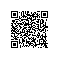# 【C语言】函数和自定义函数

函数，我之前也提到过一点点内容。其实函数是很好理解的，但是写起来又十分麻烦。

 #include main() {   printf(“Hello World!”); } 在这个C程序中，main函数是一切程序的主函数，程序必须是从main函数开始执行，到main函数结束。 函数体里面只有一个输出语句，而这个输出语句也是调用了一个printf库函数。 改为用户自定义函数形式 第1行 第2行 第3行 第4行 第5行 第6行 第7行 第8行 第9行 #include void pr1() {   printf(“Hello World!”); } main() {   pr1(); } 在这个C程序中，除了main函数外还有一个程序员自己定义的函数，函数名是pr1 整个程序的编译是从上到下。 这个程序的执行过程是先执行第6行的main函数，执行到第8行要作pr1()，此时发生了函数调用进行到第2行，然后是345，pr1函数执行到第五行结束后，返回到第8行函数调用点，继续往下执行。

pr1()函数是用户自定义函数，详细资料看《函数定义的基本形式》

这些术语要结合无参调用、有参调用、函数返回类型等来综合考虑。

1. 从函数定义的角度看，函数可分为库函数和用户定义函数两种。
(1)库函数
由Ｃ系统提供，用户无须定义， 也不必在程序中作类型说明，只需在程序前包含有该函数原型的头文件即可在程序中直接调用。在前面各章的例题中反复用到printf 、 scanf 等函数均属此类。
(2)用户定义函数
由用户按需要写的函数。对于用户自定义函数， 不仅要在程序中定义函数本身， 而且在主调函数模块中还必须对该被调函数进行类型说明，然后才能使用。
2. Ｃ语言的函数兼有其它语言中的函数和过程两种功能，从这个角度看，又可把函数分为有返回值函数和无返回值函数两种。
(1)有返回值函数
此类函数被调用执行完后将向调用者返回一个执行结果， 称为函数返回值。如数学函数即属于此类函数。 由用户定义的这种要返回函数值的函数，必须在函数定义和函数说明中明确返回值的类型。
(2)无返回值函数
此类函数用于完成某项特定的处理任务， 执行完成后不向调用者返回函数值。这类函数类似于其它语言的过程。 由于函数无须返回值，用户在定义此类函数时可指定它的返回为“空类型”， 空类型的说明符为“void”。
3. 从主调函数和被调函数之间数据传送的角度看又可分为无参函数和有参函数两种。
(1)无参函数
函数定义、函数说明及函数调用中均不带参数。 主调函数和被调函数之间不进行参数传送。 此类函数通常用来完成一组指定的功能，可以返回或不返回函数值。
(2)有参函数
也称为带参函数。在函数定义及函数说明时都有参数， 称为形式参数(简称为形参)。在函数调用时也必须给出参数， 称为实际参数(简称为实参)。 进行函数调用时，主调函数将把实参的值传送给形参，供被调函数使用。

函数体；
}

#include <stdio.h>
void pr1()
{
printf(“Hello World!”);
}
main()
{
pr1();
} ?

函数体；
}

#include <stdio.h>
void pr1(int i,int j )
{
printf(“Hello,%d,%d”,i,j);
}
main()
{
int a=5,b=2;
pr1(a,b);

#include <stdio.h>
void pr1(i,j )
int i,j;
{
printf(“Hello,%d,%d”,i,j);
}
main()
{
int a=5,b=2;
pr1(a,b);
}

1. 无返回值函数   本章上面的程序都属于是无返回值函数。
2. 有返回值函数

#include <stdio.h>
{
printf(“%d”,a+b);
}
main()
{
}

#include  <stdio.h>
{
int sum;
sum=a+b;
return sum;
}
main()
{
int c;
printf(“%d”,c);

#include <stdio.h>
{
return a+b;
}
main()
{
}

1． 函数的值只能通过return语句返回主调函数。
return 语句的一般形式为： return 表达式；

#include <stdio.h>
{
if(c==1) return a+b;
else if(c==0) return a-b;
else return 0;
}
main()
{

2. 函数值的类型和函数定义中函数的类型应保持一致。
3．不返回函数值的函数，可以明确定义为“空类型”， 类型说明符为“void”。

Ｃ语言中，可以用以下几种方式调用函数：
1.函数表达式
2.

函数调用的一般形式加上分号即构成函数语句。例如： printf ("%D",a);scanf ("%d",&b);都是以函数语句的方式调用函数。
3.

函数作为另一个函数调用的实际参数出现。 这种情况是把该函数的返回值作为实参进行传送，因此要求该函数必须是有返回值的。例如： printf("%d",max(x,y)); 即是把max调用的返回值又作为printf函数的实参来使用的。

1. 输出50行hello world

#include <stdio.h>
main()
{
int i;
for(i=0;i<50;i++)
printf(“Hello World!\n”);
}

#include <stdio.h>
void he1()
{
printf(“Hello World!\n”);
}
main()
{
int i;
for(i=0;i<50;i++)
he1();

#include  <stdio.h>
void he2()
{
int i;
for(i=0;i<50;i++)
printf(“Hello World!\n”);
}
main()
{
he2();
}
2. 素数程序：输入一个正整数，判断其是否是素数。

#include  <stdio.h>
main()
{
int n,i;
do
{
scanf("%d",&n);
}while(n<0);
for(i=2;i<n;i++)
if(n%i==0)
break;
if(i<n)  printf("No，%d 不是一个素数",n);
else    printf("Yes，%d 是一个素数",n);
}

#include <stdio.h>
void su1(int x)
{
int i;
for(i=2;i<x;i++)
if(x%i==0)
break;
if(i<x)  printf("No，%d 不是一个素数",n);
else    printf("Yes，%d 是一个素数",n);
}
main()
{
int n;
do
{
scanf("%d",&n);
}while(n<0);
su1(n);
}

#include <stdio.h>
int su2(int x)
{
int i;
for(i=2;i<x;i++)
if(x%i==0)
break;
if(i<x)  return 0;
else    return 1;
}
main()
{
int n;
do
{
scanf("%d",&n);
}while(n<0);
if(su2(n))  printf("Yes，%d 是一个素数",n);
else printf("No，%d 不是一个素数",n);

#include <stdio.h>
int su3(int x)
{
int i;
for(i=2;i<x;i++)
if(x%i==0)
break;
return x-i;
}
main()
{
int n;
do
{
scanf("%d",&n);
}while(n<0);
if(su3(n)>0)  printf("No，%d 不是一个素数",n);
else printf("Yes，%d 是一个素数",n);
}

#include<stdio.h>
int su3(int x)
{
int i;
for(i=2;i<x;i++)
if(x%i==0)
break;
return x-i;
}
main()
{
int n,m;
do
{
scanf("%d",&n);
}while(n<0);
if(su3(n)>0)  printf("No，%d 不是一个素数",n);
else printf("Yes，%d 是一个素数",n);
do
{
scanf("%d",&m);
}while(m<0);
if(su3(m))  printf("No，%d 不是一个素数",n);
else printf("Yes，%d 是一个素数",n);
}

1. 局部变量

#include<stdio.h>
void pr1(int i,int j )
{
printf(“Hello,%d,%d”,i,j);
}
main()
{
int a=5,b=2;
pr1(a,b);

pr1()函数定义了形式参数，同时也属于pr1函数的变量i,j，则此时i,j的作用域就仅仅属于pr1这个函数的范围。

printf(“%d”,i); 这样的语句是有问题的。

#include"stdio.h"
{
a=a+3;
b=b-5;
printf("a=%d,b=%d\n",a,b);
return a+b;
}
void main()
{
int a,b=2;
a=5;
printf("a=%d,b=%d\n",a,b);
printf("a=%d,b=%d",a,b);

a=5,b=2
a=8,b=-3
a+b=5
a=5,b=2

2. 全局变量

#include"stdio.h"
int sum;
{
sum=a+b;
}
void main()
{
int a=5,b=2;
printf("a+b=%d",sum);

#include"stdio.h"
int sum;
{
int sum;
sum=a+b;
}
void main()
{
int a=5,b=2;
sum=0;
printf("a+b=%d",sum);
} 程序结果：a+b=0

#include"stdio.h"
{
x=x+3;
y=y-5;
printf("x=%d,y=%d\n",x,y);
return x+y;
}
void main()
{
int a,b=2;
a=5;
printf("a=%d,b=%d\n",a,b);
printf("a=%d,b=%d",a,b);

a=5,b=2
x=8,y=-3
a+b=5
a=5,b=2

#include"stdio.h"
void change(int x,int y)
{
int temp;
printf("@2:x=%d,y=%d\n",x,y);
temp=x;
x=y;
y=temp;
printf("@3:x=%d,y=%d\n",x,y);
}
void main()
{
int a={3,2,9,6,11,4},x,y;
x=a;
y=a;
printf("@1:x=%d,y=%d\n",x,y);
change(x,y);
printf("@4:x=%d,y=%d\n",x,y);

@1:x=3,y=2
@2:x=3,y=2
@3:x=2,y=3
@4:x=3,y=2

change函数的作用是把x和y的值互换，

#include"stdio.h"
float aver(float a)
{int i;
float av,s=a;
for(i=1;i<5;i++)
s=s+a[i];
av=s/5.0;
return av;
}
void main()
{
float sco,av;
int i;
printf("\ninput 5 scores:\n");
for(i=0;i<5;i++)
scanf("%f",&sco[i]);
av=aver(sco);
printf("average score is %5.2f",av);

av=aver(sco);把sco作为实参传递给了a，此时a数组跟sco数组是一样的，而aver的功能是运算这个数组的平均分，返回这个平均分给av变量，最后输出。

#include"stdio.h"
float aver(float a[],int n)
{int i;
float av,s=a;
for(i=1;i<n;i++)
s=s+a[i];
av=s/n;
return av;
}
void main()
{
float sco,av;
int i;
printf("\ninput 5 scores:\n");
for(i=0;i<5;i++)
scanf("%f",&sco[i]);
av=aver(sco,5);
printf("average score is %5.2f",av);

#include"stdio.h"
void aver(float a[],int n)
{
int i;
for(i=0;i<5;i++)
printf("%5.1f ",a[i]);
}
void main()
{
float sco={1.2,3,4.6,5,9.6};
int i;
for(i=0;i<5;i++)
printf("%5.1f ",sco[i]);
printf("\n");
aver(sco,3);

#include"stdio.h"
void aver(float a[],int n)
{
int i;
for(i=0;i<5;i++)
printf("%5.1f ",a[i]);
}
void main()
{
float sco={1.2,3,4.6,5,9.6};
int i;
for(i=0;i<5;i++)
printf("%5.1f ",sco[i]);
printf("\n");
aver(sco,5);
}

#include"stdio.h"
void aver(float a[],int n)
{
int i;
float temp;
temp=a;
a=a;
a=temp;
for(i=0;i<5;i++)
printf("%5.1f ",a[i]);
}
void main()
{
float sco={1.2,3,4.6,5,9.6};
int i;
for(i=0;i<5;i++)
printf("%5.1f ",sco[i]);
printf("\n");
aver(sco,3);
printf("\n");
for(i=0;i<5;i++)
printf("%5.1f ",sco[i]);

1.2  3.0   4.6  5.0  9.6
3.0  1.2   4.6  5.0  9.6
3.0  1.2   4.6  5.0  9.6

#include"stdio.h"
int fx2(int x)
{
return x*x;
}
int fx1(int n)
{
return 2*n+fx2(n-1)+1;
}
void main()
{
printf("%d",fx1(3));

n!=n*(n-1)!   当n>=2时
n!=1        当n=1时
#include"stdio.h"
int digui1(int n)
{
if(n>1)
return n*digui1(n-1);
else
return 1;
}

void main()
{
int n;
do
{
printf("input n>0:n=");
scanf("%d",&n);
}while(n<0);
printf("%d",digui1(n));

1． 求岁数。

8号大两岁，以此类推，1号同学说他今年12岁，请问10号同学多少岁？

f(x)=1  x=1
#include "stdio.h"
int digui2(int n)
{
if(n>1)
return 2+digui2(n-1);
else
return 12;
}
void main()
{
printf("%d",digui2(10));
}使用钉钉扫一扫加入圈子
+ 订阅